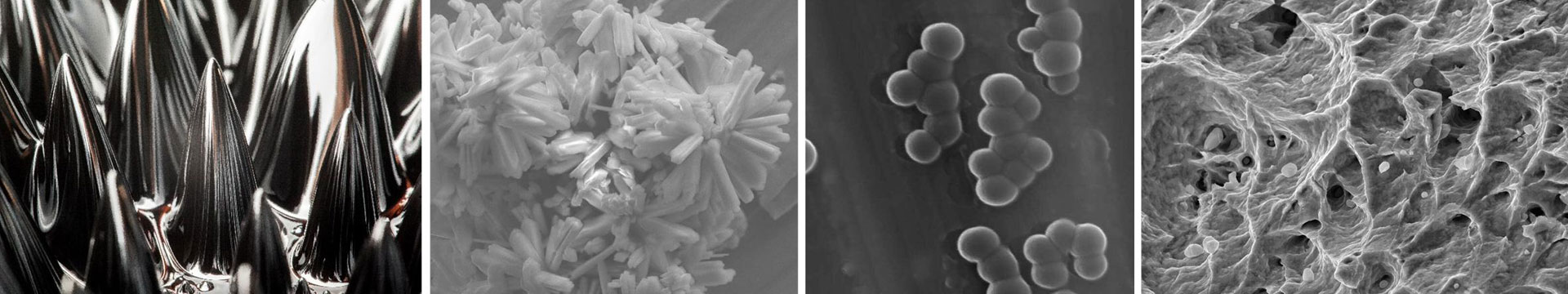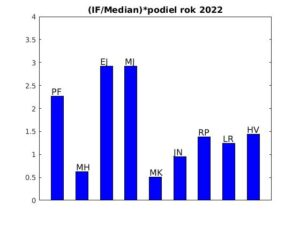•# 2022

1. P. FARKAŠOVSKÝ, Superconducting correlations in the two-dimensional Hubbard model with long-range electron hopping, Physica C: Superconductivity and its Applications 602 (2022) 1354141  [IF/MedIF(2021)=1.534/3.097=0.495] (4 pages).
2. P. FARKAŠOVSKÝ, Ferromagnetism in the Three-dimensional Hubbard Model with Generalized Type of Electron Hopping, Journal of Superconductivity and Novel Magnetism 35 (2022) 2391-2395 [IF/MedIF(2021)=1.675/3.097=0.541] (5 pages).
3. P. FARKAŠOVKÝ and L. REGECIOVÁ, Ground state and thermodynamic properties of the coupled double-Ising model: application to rare-earth tetraborides, Journal of Physics – Condensed Matter 34 (2022) 435802 [IF/MedIF(2021)=2.745/3.097=0.886] (11 pages).
4. L. REGECIOVÁ and P. FARKAŠOVSKÝ, Ground states and thermodynamics of the spin-electron model with mixed spins: Application to rare-earth tetraborides, Physica E – Low-Dimensional systems and nanostructures 143 (2022) 115396 [IF/MedIF(2021)=3.369/3.097=1.088] (6 pages).
5. L. REGECIOVÁ and P. FARKAŠOVSKÝ, The influence of double-exchange and Heisenberg interaction on magnetization processes in rare-earth tetraborides, Journal of Magnetism and Magnetic Materials 546 (2022) 168780 [IF/MedIF(2021)=3.097/3.097=1] (4 pages).
6. L.Ts. ADZHEMYAN, D.A. EVDOKIMOV, M. HNATIČ, E.V. IVANOVA, M.V. KOMPANIETS, A. KUDLIS, and D.V. ZAKHAROV, The dynamic critical exponent z for 2d and 3d Ising models from five-loop ε expansion, Physics Letters A 425 (2022) 127870 [IF/MedIF(2021)=2.707/2.688=1.007] (6 pages).
7. L.Ts. ADZHEMYAN, D.A. EVDOKIMOV, M. HNATIČ, E.V. IVANOVA, M.V. KOMPANIETS, A. KUDLIS, and D.V. ZAKHAROV, Model A of critical dynamics: 5-loop ε expansion study, Physica A 600 (2022) 127530 [IF/MedIF(2021)=3.778/2.688=1.406] (17 pages).
8. K.B. OGANESYAN, M. HNATIČ, and P. KOPČANSKY, An electron wave packet in the relativistic strophotron FEL, Laser Physics Letters 19 (2022) 016001 [IF/MedIF(2021)=1.704/2.748=0.620] (4 pages).
9. The MPD Collaboration (*M. HNATIČ, cca 500 Authors), Status and initial physics performance studies of the MPD experiment at NICA, European Physical Journal A 58 (2022) 140 [IF/MedIF(2021)=3.131/2.840=1.102] (50 pages).
10. E. JURČIŠINOVÁ and M. JURČIŠIN, Phase diagram and thermodynamic properties of the frustrated ferro-antiferromagnetic spin system on the octahedral lattice, Physica A 603 (2022) 127731 [IF/MedIF(2021)=3.778/2.688=1.406] (14 pages).
11. E. JURČIŠINOVÁ and M. JURČIŠIN, Wasp-waisted hysteresis in magnetic materials with octahedral structure: A theoretical explanation based on exactly solvable model, Physics Letters A 443 (2022) 128225 [IF/MedIF(2021)=2.707/2.688=1.007] (6 pages).
12. E. JURČIŠINOVÁ and M. JURČIŠIN, Prediction of the existence of an intermediate phase in the antiferromagnetic J1-J2system on the face-centered cubic lattice, Europhysics Letters 139 (2022) 26001 [IF/MedIF(2021)=1.958/2.688=0.728] (7 pages).
13. E. JURČIŠINOVÁ and M. JURČIŠIN, General solution of spin-1 Ising model in the effective field theory approximation: critical temperatures and spontaneous magnetization, Phase Transitions 95 (2022) 1 [IF/MedIF(2021)=1.529/3.097=0.494] (9 pages).
14. E. JURČIŠINOVÁ and M. JURČIŠIN, Influence of interaction-generated anisotropy on the antiferromagnetic spin system on the octahedral lattice, Europhysics Letters 140 (2022) 46002 [IF/MedIF(2021)=1.958/2.688=0.728] (7 pages).
15. E. JURČIŠINOVÁ and M. JURČIŠIN, Single-ion anisotropy as the source of anomalies in thermodynamic properties of magnetic spin-1/2 − 1 mixed systems on square and simple cubic lattices, Journal of Statistical Mechanics: Theory and Experiment 11 (2022) 113204 [IF/MedIF(2021)=2.234/1.520=1.470] (25 pages).
16. M. KUPKOVÁ, M. KUPKA, A. MOROVSKÁ TUROŇOVÁ, R. ORIŇÁKOVÁ, Microstructural, Mechanical and Corrosion Characteristics of Degradable PM Biomaterials Made from Copper-Coated Iron Powders, Materials 15(5) (2022) 1913 [IF/MedIF(2021)=3.748/1.864=2.011] (13 pages).
17. B.Z. KOPELIOVICH, M. KRELINA, J. NEMCHIK, and I.K. POTASHNIKOVA, Coherent photoproduction of heavy quarkonia on nuclei, Physical Review D 105 (2022) 054023 [IF/MedIF(2021)=5.407/2.840=1.904] (10 pages).
18. A. PIGAZZINI, R. PINČÁK, S. JAFARI, and C. OZEL, Emerging hyperbolic spaces and some considerations in AdS cosmology, International Journal of Geometric Methods in Modern Physics 19 (2022) 2250062 [IF/MedIF(2021)=1.873/1.520=1.232] (12 pages).
19. M. PUDLÁK, R. PINČÁK, and E. BARTOŠ, Effect of vibrational modes on electron transfer directionality: Photosynthetic reaction centers, PHYSICAL REVIEW E 105 (2022) 064408 [IF/MedIF(2021)=2.707/1.520=1.781] (7 pages).
20. U. SHEIKH, S. RASHID, C. OZEL, and R. PINČÁK, On Hosoya Polynomial and Subsequent Indices of C4C8(R) and C4C8(S) Nanosheets, Symmetry 14 (2022) 1349 [IF/MedIF(2021)=2.940/2.604=1.129] (15 pages).
21. V. V. SHUNAEV, R. PINČÁK, and O. E. GLUKHOVA, The energetical, electronic and optical properties of the intermetallic fullerene Fe@C60, Computational and Theoretical Chemistry 1214 (2022) 113784 [IF/MedIF(2021)=2.292/3.841=0.597] (5 pages).
22. H. VARGOVÁ and J. STREČKA, Conventional and inverse magnetocaloric and electrocaloric effects of a mixed spin-(1/2, 1) Heisenberg dimer, European Physical Journal Plus 137 (2022) 490 [IF/MedIF(2021)=3.758/2.688=1.398] (12 pages).
23. H. VARGOVÁ, J. STREČKA, and N. TOMAŠOVIČOVÁ, Effect of an uniaxial single-ion anisotropy on the quantum and thermal entanglement of a mixed spin-(1/2, S) Heisenberg dimer, Journal of Magnetism and Magnetic Materials 546 (2022) 168799 [IF/MedIF(2021)=3.097/3.097=1] (4 pages).
24. H. VARGOVÁ and J. STREČKA, Influence of a further-neighbour interaction on a rotating magnetoelectric effect in a coupled spin–electron model on a doubly decorated square lattice, Journal of Magnetism and Magnetic Materials 544 (2022) 168691 [IF/MedIF(2021)=3.097/3.097=1] (4 pages).#### Publikácie v registrovaných zahraničných neimpaktovaných časopisoch a zborníkoch:

1. E. A. AYRYAN, M. HNATIČ, and V. B. MALYUTIN, On the equivalence of the operator and combinatorial approaches for one-step random Markov processes, Proceedings of the National Academy of Sciences of Belarus, Рhysics and Mathematics series 58 (2022) 21 (13 pages).
2. M. KRELINA and J. NEMCHIK, Momentum transfer dependence of heavy quarkonium electroproduction, SciPost Phys. Proc. 8 (2022) 114 (6 pages).

#### Príspevky v domácich zborníkoch a konferenciách:

1. R. PINČÁK, Time travelling and parallel universes,  26th CONFERENCE OF SLOVAK PHYSICISTS: PROCEEDINGS p. 13-18, Slovak Physical Society, 2022,  ISBN 978-80-89855-19-3 (6 pages). (26. konferencia slovenských fyzikov 05.-08.09. 2022, Košice: Pozvaná prednáška)